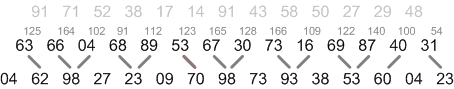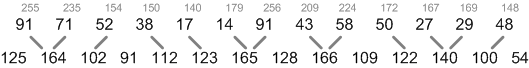# Project Euler Problem 18 in F#

2011 brings us yet another Project Euler problem to tackle: this time is Problem 18 one of the most interesting that I have solved so far:

By starting at the top of the triangle below and moving to adjacent numbers on the row below, the maximum total from top to bottom is 23.

3
7 4
2 4 6
8 5 9 3

That is, 3 + 7 + 4 + 9 = 23.

Find the maximum total from top to bottom of the triangle below:

75
95 64
17 47 82
18 35 87 10
20 04 82 47 65
19 01 23 75 03 34
88 02 77 73 07 63 67
99 65 04 28 06 16 70 92
41 41 26 56 83 40 80 70 33
41 48 72 33 47 32 37 16 94 29
53 71 44 65 25 43 91 52 97 51 14
70 11 33 28 77 73 17 78 39 68 17 57
91 71 52 38 17 14 91 43 58 50 27 29 48
63 66 04 68 89 53 67 30 73 16 69 87 40 31
04 62 98 27 23 09 70 98 73 93 38 53 60 04 23

NOTE: As there are only 16384 routes, it is possible to solve this problem by trying every route. However, Problem 67, is the same challenge with a triangle containing one-hundred rows; it cannot be solved by brute force, and requires a clever method! ;o)

## Analysis

This problem is easily solved resorting to dynamic programming, which we can loosely describe as decomposing the overall problem into easier sub-problems and combining their solutions to find the final answer.

In our case, if we consider the number associated with each node of the triangle as a cost, the overall problem can be expressed as finding the path with maximum cost from the upper vertex to the bottom row. A generic sub-problem would be the cost to reach the bottom row from a generic node at position $$i,j$$.

Given the cost $$c_{i,j}$$ of passing through a given node and the cost $$C_{i,j}$$ of reaching the bottom row from that particular node, we can define the following truth:

$$C_{i,j} = c_{i,j} + max(C_{i+1,j}, C_{i+1,j+1})$$

or, in other words, from each node $$i,j$$ the cost of reaching the bottom is equal to the cost of the node itself plus the maximum path between one of the two adjacent nodes from the row below.

Let's confirm why this is the case with the help of the following figure: consider for example the first node from the left in the fourteenth (last-but-one) row (having cost 63): from there you can move to the bottom either going to node 04 or node 62. Clearly, if we want to pick the highest cost, we need to pick 62 (and we mark this choice with a grey line). The overall cost to reach the bottom from that node is thus 63 + max (04, 62) = 125.

Let's consider now the second node from that row (66). From there, we have to choose between node 62 and 98: the highest cost path from there is thus 66 + max(62, 98) = 164. We can continue for all the nodes in the row to find the highest cost path from each node; the solution to such sub-problems is indicated in gray close to each node.Having solved all sub-problems for row 14, we can now consider the row immediately above. In the following figure, I have replaced row 14 with the solutions of the sub-problems that we had just solved; the row above is row 13. Please note how we dont care about row 15 anymore: its contribution to the overall problem is already captured in each sub-problems' solution for row 14! Hence, we proceed in a similar way to solve the sub-problems for the row 13 just as we did for row 14:We continue to follow a similar pattern moving upwards row after row, until we reach the tip of the triangle, always considering two rows at a time.

## Solution

Most of the code for this solution is needed just to initialize the two dimensional array containing the triangle. For reading convenience, I have skipped most of the uninteresting rows, but you can find the complete solution on Github.

So, we have a matrix (2D array) for the cost of each nodes and another matrix for the solutions of the sub-problems associated to each node. Trivially, the costs and the solutions coincide for the bottom row (note the handy Array2D.blit function to copy a whole row from one matrix to the other).

Then, with a loop we move backward from the last-but-one row up to the tip of the triangle calculating the sub-problems solutions as explained above.

Updated: